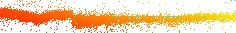```                                                 Sept.21,'99
11/27/99

```

### ACIS-S FI Chips: CTI Criteria for HETG Use

```        -----------------------------------

- FI devices need to detect photons above 0.80 keV.

o For the MEG spectra, the S1 chip takes over from FIs
at ~0.85 keV.  For HEG spectra, S5 covers down
to ~0.8 keV before falling off the chip.  There is a
bright/useful Fe line at 0.826 keV (15.012 A).
So, all things considered, FI performance to 0.8 keV
is highly desirable.

```http://space.mit.edu/HETG/flight/obs/Obs62538/
```
- FI devices need energy resolution E/dE ~ 2 (TBR).

o As the HR1099 point source plot above shows, excluding
the 2nd and higher orders in the HEG and MEG spectra
can be done with relatively crude E/dE.  The 2nd and
higher orders need only be excluded to some fraction of
the first order flux.  In order to be able to estimate
2nd order effects we need to i) be able to measure 2nd
order distribution intensity and ii) calibrate 2nd order
relative efficiency.  Even crude ACIS-S energy resolution
will go a long way in helping with order sorting.

o Assuming the rough relation:

E / dE ~=~ 1.7 / (CTI x CHIPY)

and requiring an E/dE value of 2.0 at CHIPY ~ 850 gives a
maximum OK value of CTI of:  10 x 10^-4 .

o Examples of PHA distributions relevant to order sorting
are shown here.  These are essentially a vertical slice
through the Grating-E - ACIS-E plots.  First, second
and third orders are generally visible.  Gaussians with
E/dE of 10 and 2 at E_peak, 2E_peak, and 3E_peak are over-
plotted for reference.  The ACIS Energy is taken directly
from the REQUEST 187 Level 1 events ENERGY value (uncorrected.)
The data are from the Day 258 HR1099 observation as in plot above.
```
```
- FI devices need to be calibrated in QE-as-used to 10% (TBR).

o Some HETGS science relies on accurate flux values
and ratios.  To be useful, the effective QE of the
FI devices must be known to 10% or better (TBR).

- These criteria apply to specific regions of the FI devices.

o The full extent (1024 rows) of the s-array chips are not
required by HETG for a point-like source.  The criteria
apply to photons arriving on the HETG "X" when zero-order
is placed in YCHIP = row 300 (TBR).  This reduces parallel
transfers to the framestore area; maximum transfers is then
less than 600 pixels.
[ row 300  ~=
250 +     dY zero-order to HEG 0.826 keV line
16 +     8" dither amplitude
30       15" pointing accuracy               ]

- - - end - - -
```